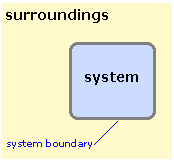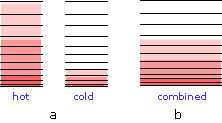## Unit 6 Study Guide: Thermochemistry and Thermodynamics

### 6a: Define temperature.

While we talk about temperature nearly every day, it has a specific definition central to thermodynamics.

• Define temperature.

To understand temperature, first we need to define different types of energy.

Kinetic energy is the energy of motion, related to an object’s mass and velocity, or speed.

Potential energy is the energy an object has based on its location. If an object is in a location where it is subject to a restoring force, such as gravity, it has potential energy. Gravity is an example of a restoring force. Because energy is conserved, potential energy can be converted into other types of energy.Chemical energy is the energy stored between the chemical bonds of molecules. As we have discussed above, chemical energy relates to the potential electrostatic energy forces that exist between electrons and the atomic nuclei.

Thermal energy is a microscopic version of kinetic energy. When an event involving energy transfer occurs (for example, a chemical reaction, a ball being dropped from a high surface), some of the energy is dispersed into the surrounding atoms or molecules in a random manner. This is thermal energy. Temperature is the measure of thermal energy.

### 6b: Define heat and state its units.

In thermodynamics, we use precise definitions for many words we use in our common language. It is important to know these definitions and use these words properly when describing thermodynamics.

• What is the definition of heat?
• What units are used to describe heat?

In thermodynamics, we define heat as the process where an object acquires or loses energy because it has a different temperature than its surroundings. It is important to note that thermal energy can only flow from high temperature to low temperature.

Heat has the same units as other energies. The most common unit used in chemistry is the joule (J). We also use the energy unit calorie (cal). Note that one cal = 4.184 J.

Review a listing of different energy units in the table in Section 2: Energy Scales and Units, in Chemical Energetics.

### 6c: Define and perform enthalpy change, enthalpy of reaction, enthalpy of combustion, and enthalpy of formation calculations.

• Define enthalpy.
• Calculate the enthalpy change for a given system.
• Determine the enthalpy of reaction for a given reaction.
• Determine the enthalpy of combustion for a given system.
• Calculate enthalpy using standard enthalpy of formation.

Before we can define enthalpy, you need to understand how to describe a thermodynamics problem. When discussing thermodynamics, we think of the system and the surrounding.

The system describes what we are interested in. The surroundings describe everything around the system the system may interact with. A system is closed when a boundary prevents the matter in the system from entering the surroundings. In an open system, matter can flow from the system to the surroundings.We define enthalpy (ΔH) as the heat change in a system at constant pressure (note that Δ is the Greek symbol delta which means “change,” so you can read ΔH as a change in heat).

We can express enthalpy mathematically as:
ΔH ≡ qP = ΔU + PΔV .

You must use this equation to calculate ΔH for a given system. Note that to calculate ΔH you will probably be given ΔU, P (pressure), and change of volume in the system.

Review Equation (4-3) under the heading, Enthalpy Hides Work and Saves it Too!, in The First Law of Thermodynamics.

For this equation:

Make sure your units match so your final answer is in the same energy units. You may need to employ a unit conversion factor to convert the work term to an energy unit.

Review how to calculate enthalpy and use a unit conversion factor to ensure proper units in Problem Example 3 in The First Law of Thermodynamics.

Just as Problem Example 3 demonstrates how to calculate enthalpy for a simple system, we can also calculate enthalpy for a chemical reaction. We can write the thermochemical equation that describes the process, including the substances and their states.

For example, the thermochemical equation for boiling water at its normal boiling point is:

H2O (l, 373 K, 1 atm) → H2O (g, 373 K, 1 atm) ΔH = 40.7 kJ mol–1

Here, ΔH is known as the enthalpy of vaporization of water. It describes the change in heat at constant pressure for the vaporization process.

To determine the enthalpy of reaction, we use the following equation:

ΔH = H products – Hreactants

Review Section 2: Standard Enthalpy of Formation, in The First Law of Thermodynamics.

Here,

Hproducts represents the enthalpy of products. Hreactants represents the enthalpy of the reactants.

The question remains, how do we know the H products and H reactants?

We use the standard enthalpies of formation for each of the reactants and products. The standard enthalpy of formation for a compound is the heat associated with the formation of one mole of the compound from its elements in their standard states.

For example, the following equation describes the standard enthalpy of formation of water:

H2(g) + ½ O2(g)→ H2O(l) ΔH = –286 kJ

You can find tables of standard enthalpies of formation in chemistry texts and online.

Consequently, we can write the equation for enthalpy of reaction as:

ΔH°reaction = Σ ΔHf °products Σ ΔHf °reactants

Review Equation (2-1) in Thermochemistry and Calorimetry.

To calculate the enthalpy of reaction, add the sum of the standard enthalpies of formation of the products, and subtract the sum of the standard enthalpies of the reactants.

By definition, combustion is the burning of a substance in oxygen. Hence, the enthalpy of combustion is the enthalpy change associated with burning a substance in oxygen.

Combustion is a highly exothermic (or heat releasing) process so these are easily measurable quantities. The enthalpy of combustion for many compounds are readily available in texts and online, just like the enthalpies of formation. Consequently, we can use enthalpy of combustion in the same way we use enthalpy of formation to calculate enthalpy of reaction.

### 6d: Define entropy.

• What are spontaneous processes?
• Define entropy.

Processes that proceed in a definite direction without needing a “push” are called spontaneous processes.

The direction of a spontaneous process is determined by the degree of change in the disorder around the system, not by changes in heat.

Changes that increase disorder are spontaneous; changes that decrease disorder are non-spontaneous. In a chemical process, a system can occupy many different levels or states of energy. A spontaneous process increases the number of states the system occupies, and consequently increases the disorder of the system.

For example, let’s look at heat flow. We stated above that heat flows from hot to cold. The hot state has more energy, and occupies more energy levels, than the cold system. When heat flows from hot to cold, as in this figure, energy is distributed, which increases the disorder within the cold system.Based on this concept, entropy S, measures how much thermal energy spreads in a chemical or physical change. Spreading energy can be based on the available space, such as a gas that expands, or on the energy states available, such as in the above heat flow example.

### 6e: Describe the driving force of a chemical reaction and relate it to Gibbs free energy.

• Define Gibbs Free Energy.
• Use Gibbs Free Energy to explain the driving force of a chemical reaction.

We can express Gibbs Free Energy (ΔG) mathematically as ΔG = ΔH – T ΔS

(ΔH represents the enthalpy change of the reaction, T represents temperature, and ΔS represents the change in entropy of the system.)

Review Equation (4-2) in Free Energy: The Gibbs Function (Gibbs Energy).

Gibbs Free Energy combines important information from enthalpy and entropy to determine the extent and direction of a chemical change. The sign of ΔG determines the direction of spontaneity of a chemical reaction.

Review this chart:

 ΔG < 0 Spontaneous to the right (products) ΔG > 0 Spontaneous to the left (reactants) ΔG = 0 System at equilibrium

Review details on the interplay between the enthalpy and entropy terms in the Gibbs Free Energy equation in cases one to four in Section 2: Gibbs Energy and Chemical Change, in Free Energy: The Gibbs Function (Gibbs Energy).

### 6f: define Hess' law and state its functions.

• Define state function.
• Define Hess’ Law and explain how it is used.

State functions are mathematical functions that always give the same result, regardless of the steps taken to get there. Many thermodynamic functions are state functions, including enthalpy.

Imagine you want to take an elevator from the second to fifth floor. The elevator could take you directly to the fifth floor, or take you down to the first floor and up to five. The net change remains the same: you went from the second to the fifth floor, regardless of the path you took to get there.

Hess’ Law states that the enthalpy of a chemical reaction is constant, regardless of the path taken. In other words, the net enthalpy of a reaction will always be the same regardless of how many steps it took for the net reaction to occur.

Hess’ Law is extremely useful because it allows us to calculate the enthalpy for almost any reaction based on known quantities. Since we have calculated the standard enthalpies of formation for most compounds used in chemical reactions, we can determine the enthalpy of reaction.

Review a description Hess’ Law from the beginning of Section 3: Hess’ Law and Thermochemical Calculations, in Thermochemistry and Calorimetry.

### 6g: use Hess' law to solve thermodynamic problems.

• Use Hess’ law to determine the enthalpy of a reaction from enthalpies of formation and/or combustion.

To use Hess’ law, you must find enthalpy of formation and/or combustion reactions that can be added up to create the chemical reaction you are interested in.

• If you flip the enthalpy of formation/combustion reaction, you must flip the sign of ΔHf.
• If you multiply a reaction by a coefficient to get the right number of reactants and products, you must multiply ΔHf by the same coefficient.
• After you find the set of enthalpy of formation/combustion reactions that add up to the reaction you want and you alter the ΔHf terms appropriately, you simply add the ΔHf terms up to get ΔHreaction.

Review how to use Hess’ law in Problem Example 1 in Thermochemistry and Calorimetry.

Review additional examples of using Hess’ law in Hess' Law of Constant Heat Summation: Using Two Equations and their Enthalpies.

### Unit 6 Vocabulary

• Calorie (cal)
• Chemical energy
• Closed system
• Combustion
• Constant pressure
• Enthalpy
• Enthalpy of combustion
• Enthalpy of formation
• Enthalpy of reaction
• Enthalpy of vaporization
• Entropy
• Entropy S
• Exothermic
• Gibbs Free Energy
• Gravity
• Heat
• Hess’ Law
• Internal energy
• Joule (J)
• Kinetic energy
• Nonspontaneous process
• Open system
• Potential energy
• Restoring force
• Spontaneous process
• Standard enthalpy of formation
• State function
• Surroundings
• System
• Temperature
• Thermal energy
• Thermochemical equation
• Thermodynamics
Last modified: Wednesday, July 17, 2019, 6:06 PM